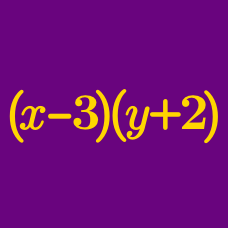Algebra

# Combining Like Terms

What is the coefficient of $x^ {4}$ in the following polynomial:

$-6 x^ {4} + 4 x^3 + 15 x^{4} + 4 x^{15} ?$

After combining like terms, what is the sum of the coefficients of the $xy$ and $x$ terms in the polynomial $3x+7xy+25y+11x-3xy?$

After combining like terms, what is the sum of all coefficients of the polynomial $2x-(x-12y-(6x-5y))?$

For example, the polynomial $2x + 3y + 2$ has coefficients of $2, 3$ and $2$, and the sum of all coefficients is $2 + 3 + 2 = 7$.

Rewrite the following expression in the form $ax + by$, and determine the sum of $a$ and $b$: $5x-(4x+3y-(2x-y))+63y.$

Given $f(x) = 8x + 5$ and $g(x) = 5x + 12$, what is the value of the constant term in $f(x) + g(x)$?

×

Problem Loading...

Note Loading...

Set Loading...